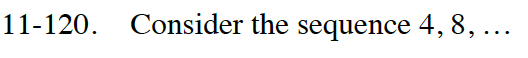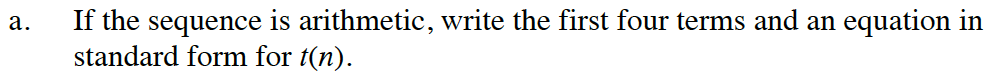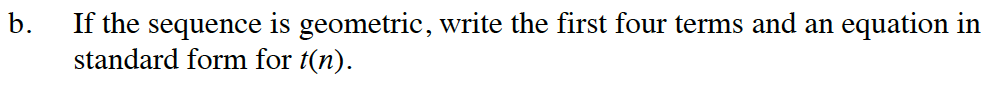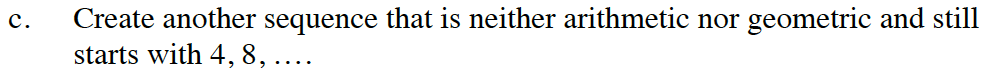Home > CC4 > Chapter 11 > Lesson 11.2.6 > Problem11-120

11-120.
1. Consider the sequence 4, 8, … Homework Help ✎

1. If the sequence is arithmetic, write the first four terms and an equation in standard form for t(n).

2. If the sequence is geometric, write the first four terms and an equation in standard form for t(n).

3. Create another sequence that is neither arithmetic nor geometric and still starts with 4, 8, ….What is the sequence generator if addition is being used to get from one term to the next?

t(n) = 4 + 4(n − 1)What is the sequence generator if multiplication is being used to get from one term to the next?

t(n) = 4(2)(n−1)There are many sequences of numbers that satisfy these conditions.
Ensure that whichever sequence you choose, it is neither arithmetic nor geometric.